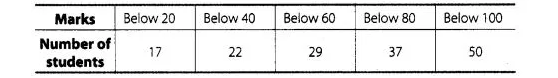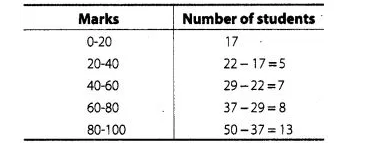# Given below is a cumulative frequency distribution

Question:

Given below is a cumulative frequency distribution showing the marks secured by 50 students of a classForm the frequency distribution table for the data.

Solution:

Here, we observe that, 17 students have scored marks below 20 i.e., it lies between class interval 0-20 and 22 students have scored marks below

40, so 22 -17 = 5 students lies in the class interval 20-40 continuing in the same manner, we get the complete frequency distribution table for given

data.